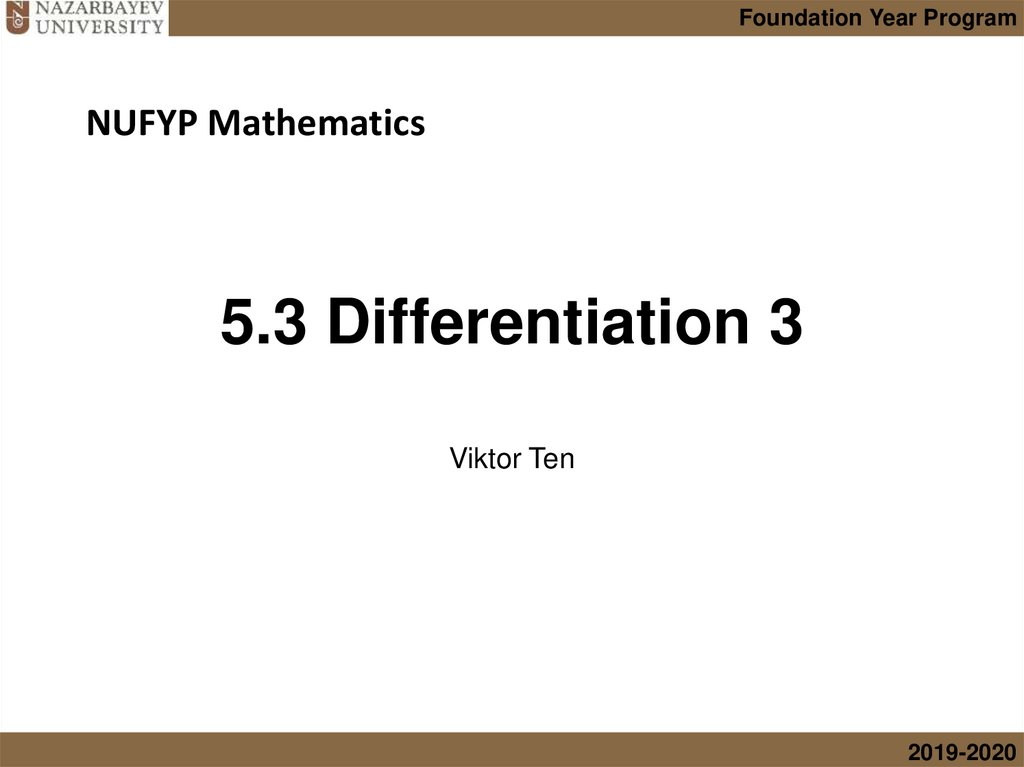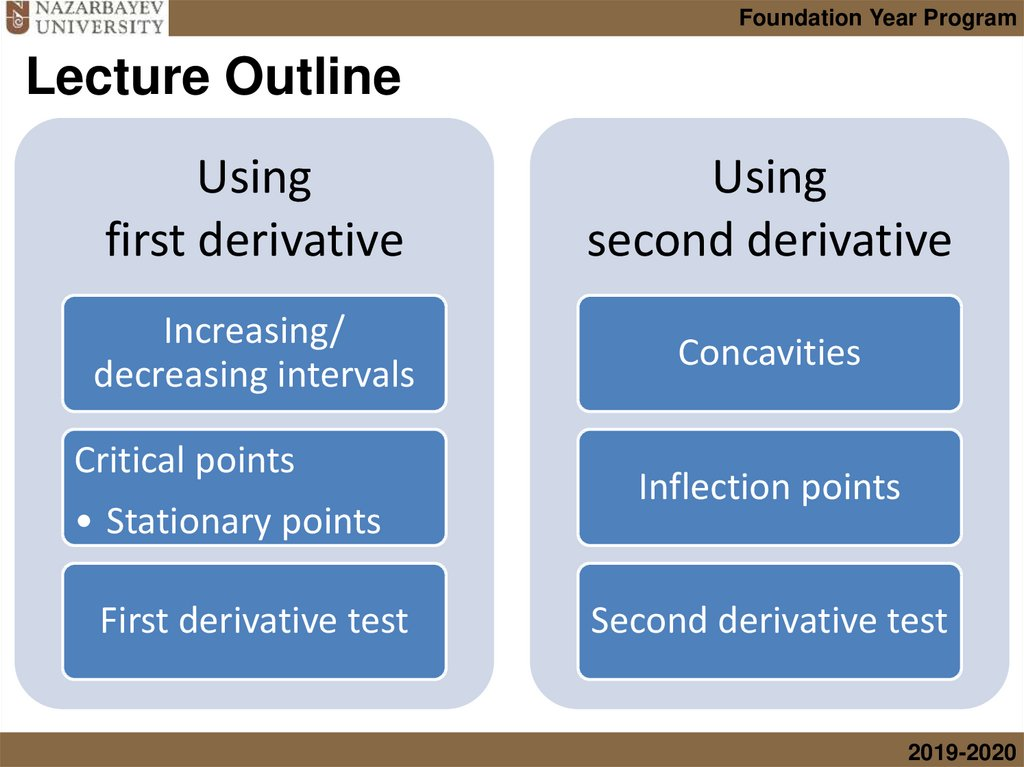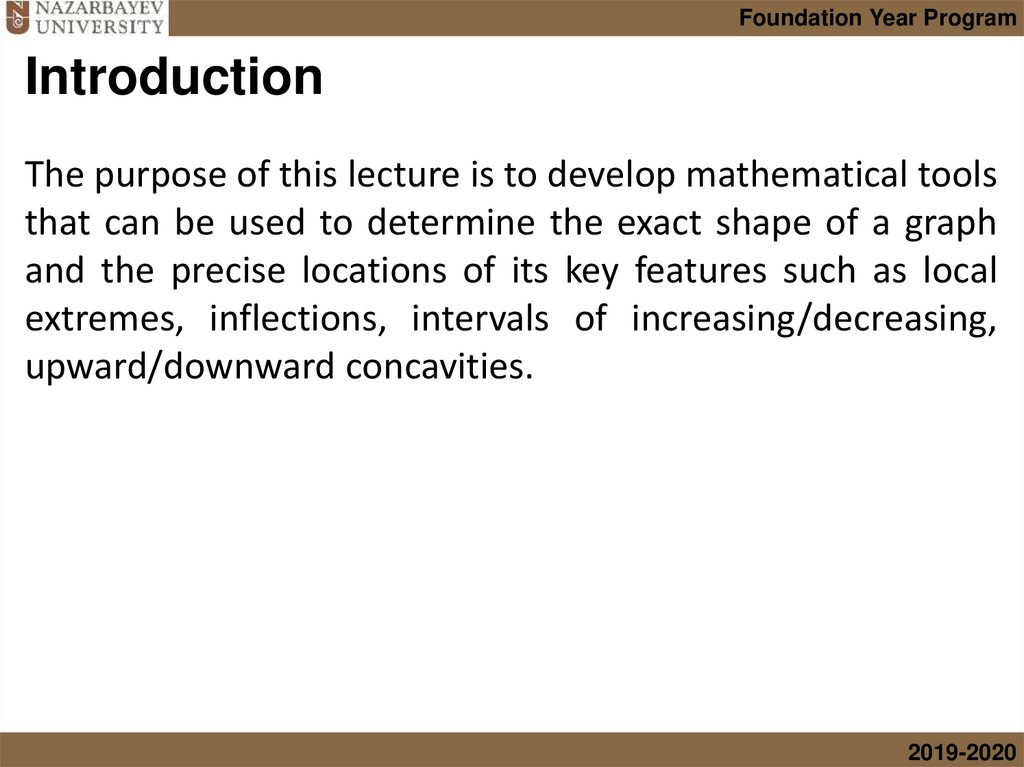# Using first derivative. Using second derivative

## 1. 5.3 Differentiation 3

Foundation Year Program
NUFYP Mathematics
5.3 Differentiation 3
Viktor Ten
2019-2020

## 2. Lecture Outline

Foundation Year Program
Lecture Outline
Using
first derivative
Using
second derivative
Increasing/
decreasing intervals
Concavities
Critical points
• Stationary points
First derivative test
Inflection points
Second derivative test
2019-2020

## 3. Introduction

Foundation Year Program
Introduction
The purpose of this lecture is to develop mathematical tools
that can be used to determine the exact shape of a graph
and the precise locations of its key features such as local
extremes, inflections, intervals of increasing/decreasing,
upward/downward concavities.
2019-2020

## 4. Increasing and decreasing functions

Foundation Year Program
Increasing and decreasing functions
The terms increasing, decreasing, and constant are used to describe
the behavior of a function as we travel left to right along its graph.
The function in the figure can be described as
increasing to the left of